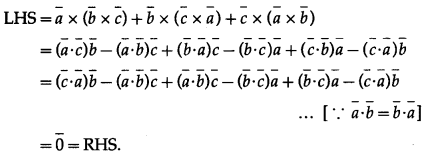Balbharti 12th Maharashtra State Board Maths Solutions Book Pdf Chapter 5 Vectors Ex 5.5 Questions and Answers.

## Maharashtra State Board 12th Maths Solutions Chapter 5 Vectors Ex 5.5

Question 1.
Find $$\bar{a}$$∙($$\bar{b}$$ × $$\bar{c}$$), if $$\bar{a}$$ = $$3 \hat{i}-\hat{j}+4 \hat{k}$$, $$\bar{b}$$ = $$2 \hat{i}+3 \hat{j}-\hat{k}$$ and $$\bar{c}$$ = $$-5 \hat{i}+2 \hat{j}+3 \hat{k}$$
Solution:
$$\bar{a}$$∙($$\bar{b}$$ × $$\bar{c}$$) = $$\left|\begin{array}{rrr} 3 & -1 & 4 \\ 2 & 3 & -1 \\ -5 & 2 & 3 \end{array}\right|$$
= 3(9 + 2) + 1 (6 – 5) + 4(4 + 15)
= 33 + 1 + 76
= 110.

Question 2.
If the vectors $$3 \hat{i}+5 \hat{k}, 4 \hat{i}+2 \hat{j}-3 \hat{k}$$ and $$3 \hat{i}+\hat{j}+4 \hat{k}$$ are to co-terminus edges of the parallelo piped, then find the volume of the parallelopiped.
Solution: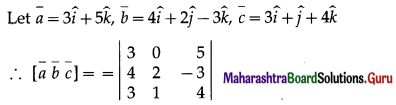= 3(8 + 3) – 0(16 + 9) + 5(4 – 6)
= 33 – 0 – 10 = 23
∴ volume of the parallelopiped = $$[\bar{a} \bar{b} \bar{c}]$$
= 23 cubic units.Question 3.
If the vectors $$-3 \hat{i}+4 \hat{j}-2 \hat{k}, \hat{i}+2 \hat{k}$$ and$$\hat{i}-p \hat{j}$$ are coplanar, then find the value of p.
Solution: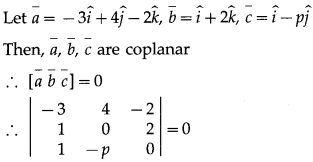∴ -3(0 + 2p) – 4(0 – 2) – 2(-p – 0) = 0
∴ -6p + 8 + 2p = 0
∴ -4p = -8
P = 2.

Question 4.
Prove that :
(i) $\bar{a} \bar{b}+\bar{c} \bar{a}+\bar{b}+\bar{c}$ = 0
Solution: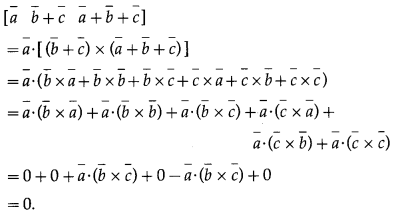(ii) ($$\bar{a}$$ – $$2 \bar{b}$$ – $$\bar{c}$$)∙[($$\bar{a}$$ – $$\bar{b}$$) × $$\bar{a}$$ – $$\bar{b}$$ – $$\bar{c}$$] = 3[$$\bar{a}$$ – $$\bar{b}$$ – $$\bar{c}$$]
Question is modified.
($$\bar{a}$$ – $$2 \bar{b}$$ – $$\bar{c}$$) [($$\bar{a}$$ – $$\bar{b}$$) × $$\bar{a}$$ – $$\bar{b}$$ – $$\bar{c}$$] = 3[$$\bar{a}$$ $$\bar{b}$$ $$\bar{c}$$]
Solution: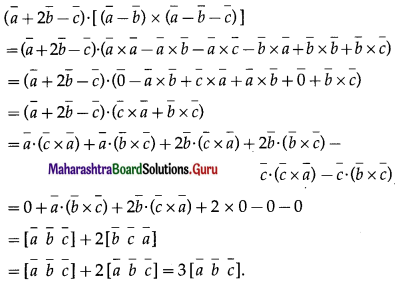Question 5.
If $$\bar{c}$$ =3$$\bar{a}$$ – 2$$\bar{b}$$ prove that [$$\bar{a}$$ $$\bar{b}$$ $$\bar{c}$$] = 0
Solution:
We use the results :$$\bar{b}$$ × $$\bar{b}$$ = 0 and if in a scalar triple product, two vectors are equal, then the scalar triple product is zero.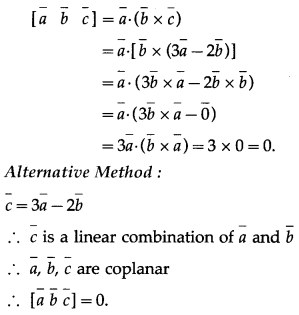Question 6.
If u = $$\hat{\mathbf{i}}-2 \hat{\mathbf{j}}+\hat{k}$$, $$\bar{v}$$ = $$3 \hat{\mathbf{i}}+\hat{k}$$ and $$\bar{w}$$ = $$\hat{\mathrm{j}}-\hat{\mathrm{k}}$$ are given vectors, then find
(i) [$$\bar{u}$$ + $$\bar{w}$$]∙[($$\bar{w}$$ × $$\bar{r}$$) × ($$\bar{r}$$ × $$\bar{w}$$)]
Question is modified.
If $$\bar{u}$$ = $$\hat{\mathbf{i}}-2 \hat{\mathbf{j}}+\hat{k}$$, $$\bar{r}$$ = $$3 \hat{\mathbf{i}}+\hat{k}$$ and $$\bar{w}$$ = $$\hat{\mathrm{j}}-\hat{\mathrm{k}}$$ are given vectors, then find [$$\bar{u}$$ + $$\bar{w}$$]∙[($$\bar{u}$$ × $$\bar{r}$$) × ($$\bar{r}$$ × $$\bar{w}$$)]
Solution: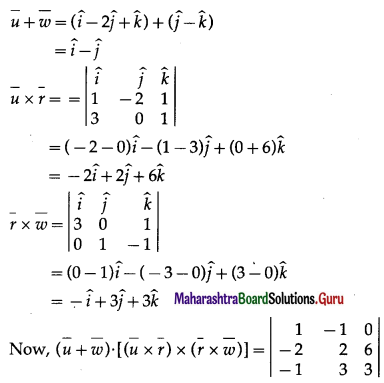= 1(6 – 18) + 1 (-6 + 6) + 0
= -12 + 0 + 0 = -12.

Question 7.
Find the volume of a tetrahedron whose vertices are A( -1, 2, 3) B(3, -2, 1), C (2, 1, 3) and D(-1, -2, 4).
Solution:
The position vectors $$\bar{a}$$, $$\bar{b}$$, $$\bar{c}$$ and $$\bar{d }$$ of the points A, B, C and D w.r.t. the origin are $$\bar{a}$$ = $$-\hat{i}+2 \hat{j}+3 \hat{k}$$, $$\bar{b}$$ = $$3 \hat{i}-2 \hat{j}+\hat{k}$$, $$\bar{c}$$ = $$2 \hat{i}+\hat{j}+3 \hat{k}$$ and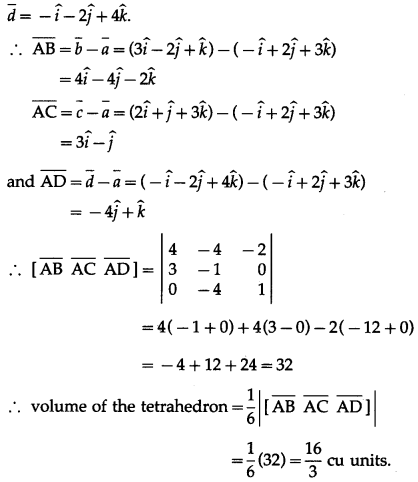Question 8.
If $$\bar{a}$$ = $$\hat{i}+2 \hat{j}+3$$, $$\bar{b}$$ = $$3 \hat{i}+2 \hat{j}$$ and $$\bar{c}$$ = ,$$2 \hat{i}+\hat{j}+3$$ then verify that $$\bar{a}$$ × ($$\bar{b}$$ × $$\bar{c}$$) = ($$\bar{a}$$ ⋅ $$\bar{c}$$)$$\bar{b}$$ – ($$\bar{a}$$ ⋅ $$\bar{b}$$)$$\bar{c}$$
Solution: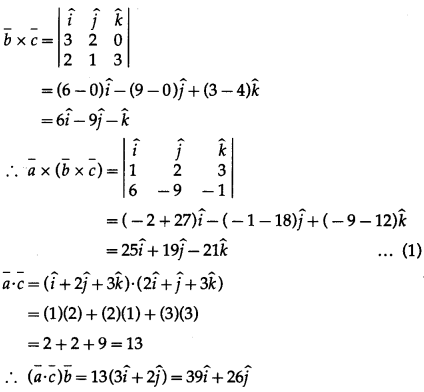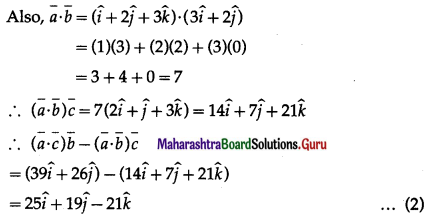From (1) and (2), we get
$$\bar{a}$$ × ($$\bar{b}$$ × $$\bar{c}$$) = ($$\bar{a}$$ ⋅ $$\bar{c}$$)$$\bar{b}$$ – ($$\bar{a}$$ ⋅ $$\bar{b}$$)$$\bar{c}$$

Question 9.
If, $$\bar{a}$$ = $$\hat{i}-2 \hat{j}$$, $$\bar{b}$$ = $$\hat{i}+2 \hat{j}$$ and $$\bar{c}$$ =$$2 \hat{i}+\hat{j}-2$$ then find
(i) $$\bar{a}$$ × ($$\bar{b}$$ × $$\bar{c}$$)
Solution: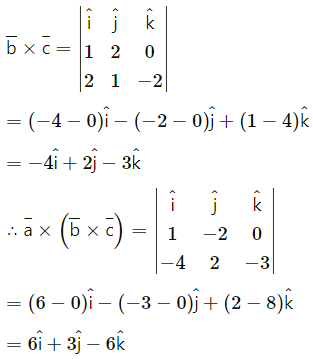(ii) ($$\bar{a}$$ × $$\bar{b}$$) × $$\bar{c}$$ Are the results same? Justify.
Solution: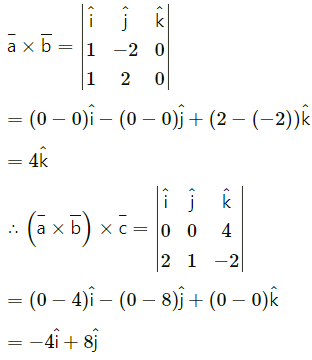$$\bar{a}$$ × ($$\bar{b}$$ × $$\bar{c}$$) ≠ ($$\bar{a}$$ × $$\bar{b}$$) × $$\bar{c}$$Question 10.
Show that $$\bar{a}$$ × ($$\bar{b}$$ × $$\bar{c}$$) + $$\bar{b}$$ × ($$\bar{c}$$ × $$\bar{a}$$) + $$\bar{c}$$ × ($$\bar{a}$$ × $$\bar{b}$$) = 0
Solution: